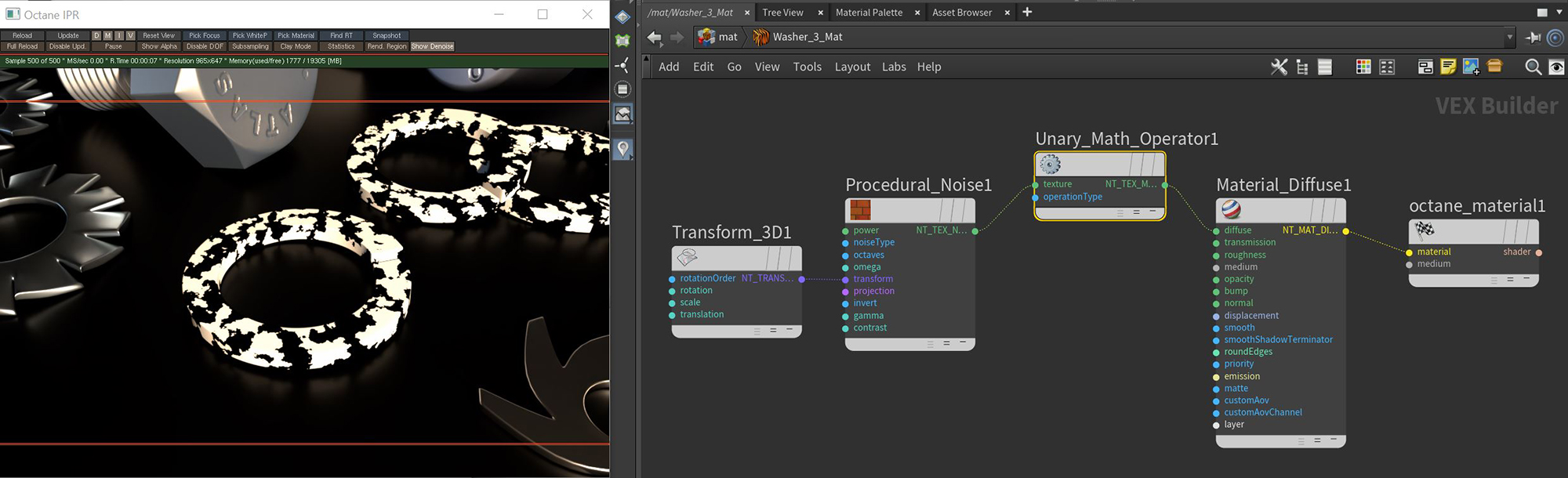# Unary Math Operator

The Unary Math Operation node takes a single input and performs a selected mathematical calculation on the data (figure 1).Figure 1: A Noise texture's contrast has been increased using the Round operation available with the Unary Math Operation node

## Unary Math Operation Parameters

Argument - The argument for the operation.

Operation - The calculation to be performed on the arguments.

• Absolute Value
• Exponential [2^x]
• Exponential [e^x]
• Exponential [e^x-1]
• Fraction
• Inverse square root
• Invert
• Logarithm Base 10
• Logarithm Base 2
• Logarithm Base e
• Negate
• Reciprocal
• Sign
• Square Root
• There are two Conversion operations:
• Degrees
• There are four rounding operations:
• Round
• Round Down
• Round Up
• Truncate
• There are nine trigonometric operations:
• Arc Cosine
• Arc Sine
• Arc Tangent
• Cosine
• Hyperbolic Cosine
• Hyperbolic Sine
• Hyperbolic Tangent
• Sine
• Tangent